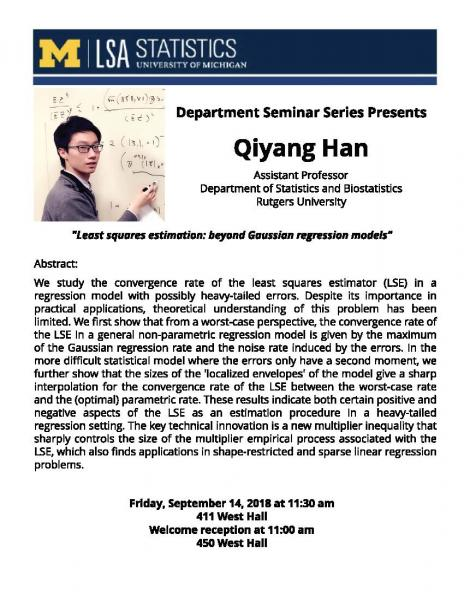Search: {{\$root.lsaSearchQuery.q}}, Page {{\$root.page}}

{{item.title}}
 {{item.snippet}}

Statistics Department Seminar Series: Qiyang Han, Assistant Professor, Department of Statistics, Rutgers University

"Least squares estimation: beyond Gaussian regression models"Friday, September 14, 2018
11:30 AM-1:00 PM
411 West Hall Map
We study the convergence rate of the least squares estimator (LSE) in a regression model
with possibly heavy-tailed errors. Despite its importance in practical applications,
theoretical understanding of this problem has been limited. We first show that from a
worst-case perspective, the convergence rate of the LSE in a general non-parametric regression model is given by the maximum of the Gaussian regression rate and the noise rate induced by the errors. In the more difficult statistical model where the errors only have a second moment, we further show that the sizes of the 'localized envelopes' of the model give a sharp interpolation for the convergence rate of the LSE between the worst-case rate and the (optimal) parametric rate.
These results indicate both certain positive and negative aspects of the LSE as an estimation procedure in a heavy-tailed regression setting. The key technical innovation is a new multiplier inequality that sharply controls the size of the multiplier empirical process associated with the LSE, which also finds applications in shape-restricted and sparse linear regression problems.
Building: West Hall Workshop / Seminar seminar Happening @ Michigan from Department of Statistics Seminar Series, Department of Statistics